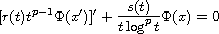Electron. J. Diff. Equ., Vol. 2016 (2016), No. 120, pp. 1-12.

### Non-oscillation of periodic half-linear equations in the critical case Petr Hasil, Michal Vesely

Abstract:
Recently, it was shown that the Euler type half-linear differential equationswith periodic coefficients r and s are conditionally oscillatory and the critical oscillation constant was found. Nevertheless, the critical case remains unsolved. The objective of this article is to study the critical case. Thus, we consider the critical value of the coefficients and we prove that any considered equation is non-oscillatory. Moreover, we analyze the situation when the periods of coefficients r and s do not need to coincide.

Submitted September 3, 2015. Published May 13 2016.
Math Subject Classifications: 34C10, 34C15.
Key Words: Half-linear equations; Prufer angle; oscillation theory; conditional oscillation; oscillation constant.

Show me the PDF file (239 KB), TEX file for this article.Petr Hasil Department of Mathematics and Statistics Faculty of Science, Masaryk University Kotlarska 2, CZ 611 37 Brno, Czech Republic email: hasil@mail.muni.cz Michal Vesely Department of Mathematics and Statistics Faculty of Science, Masaryk University Kotlarska 2, CZ 611 37 Brno, Czech Republic email: michal.vesely@mail.muni.cz